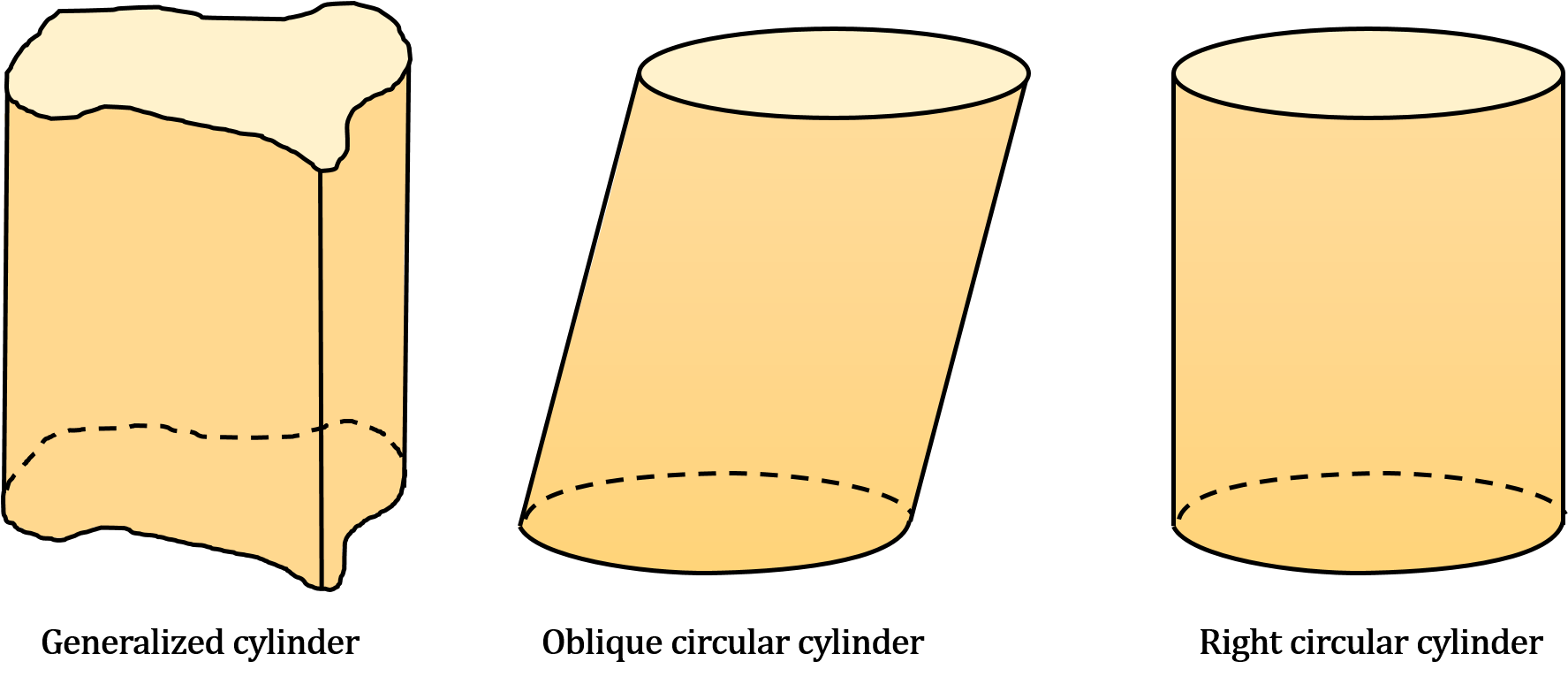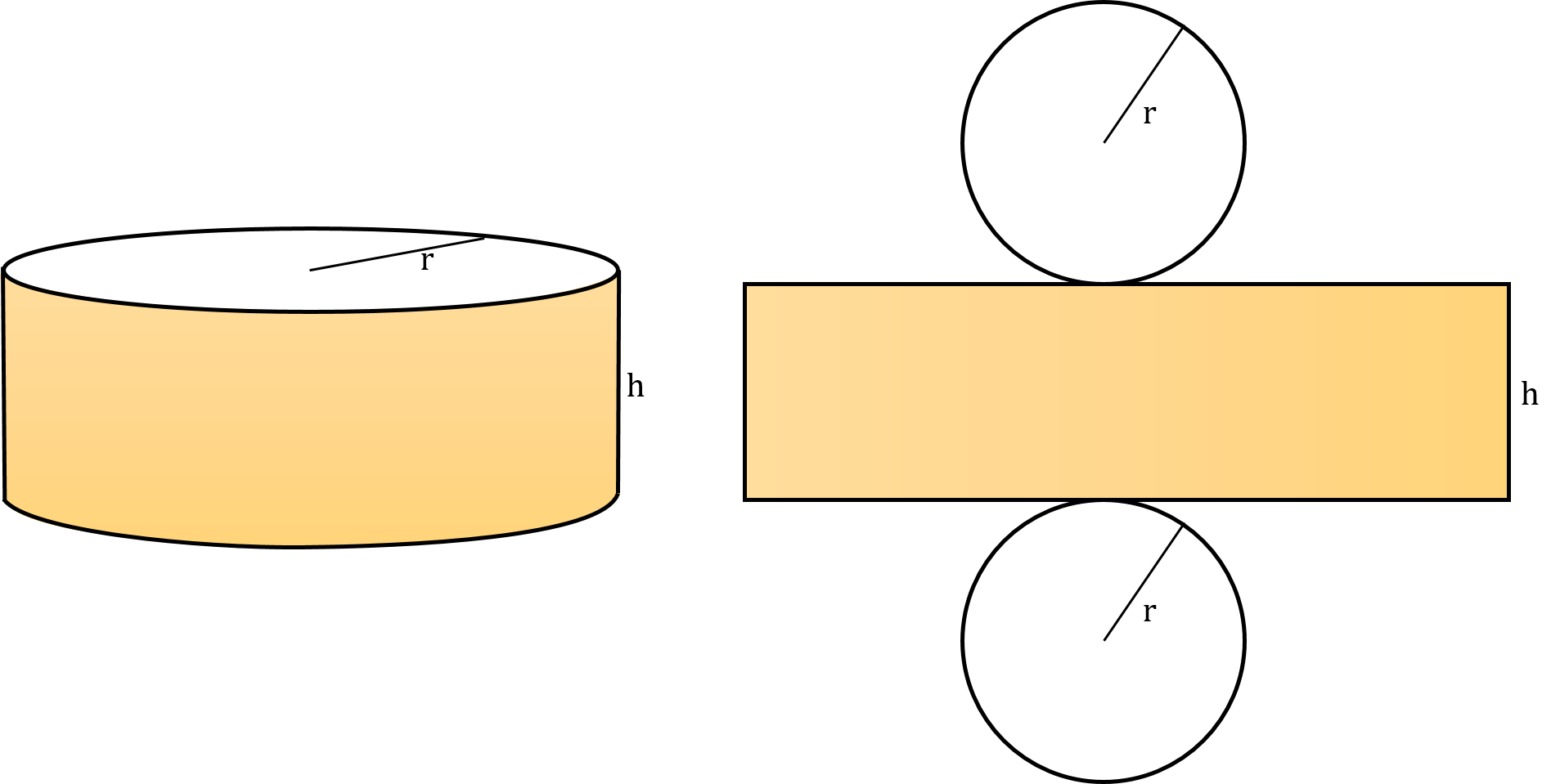# Surface Area of a Cylinder Calculator

Created by Dominik Czernia, PhD
Reviewed by Bogna Szyk and Adena Benn
Last updated: Feb 13, 2023

This surface area of a cylinder calculator is a handy tool that quickly finds all of the three types of surface areas:

• The base surface area of a cylinder;
• The lateral surface area of a cylinder; and
• The total surface area of a cylinder.

A cylinder is a three-dimensional solid that consists of two congruent surfaces (bases) and one lateral surface. Although cylinders may take many forms, the term cylinder usually means the right circular cylinder. Our surface area of a cylinder calculator is dedicated to this type of cylinder. The cylinder is right when one of its bases lies exactly above the other base and oblique if it doesn't. It is worth mentioning that the base of a cylinder can be any plain, closed surface, e.g., a circular cylinder has a circular base, and a rectangular cylinder has a rectangular baseKeep reading if you want to learn what is the surface area of a cylinder formula and how to find the surface area of a cylinder. You may also want to estimate other parameters of a cylinder – just check out our right cylinder calculator!

## How to find the surface area of a cylinder?

To estimate the surface area of a cylinder, you need to visualize it as a net. It is as if you open the cylinder just like a carton box and flatten it out. Use your imagination! And what will you get? The answer is that a right circular cylinder consists of two circles and one rectangle, as you can see in the figure below.Therefore, the base surface area of a cylinder equals two times the area of a circle with the radius r, and the lateral surface area of a cylinder is the area of a rectangle. The first side of this rectangle is the height of the cylinder h, and the second is the circumference of the base equal to 2 × π × r, according to the circumference calculator.

## What's the surface area of a cylinder formula?

Now that we know how to find the surface area of a cylinder let's derive appropriate formulas for the surface area of a right circular cylinder. To calculate the base surface area, you need to compute the area of a circle with the radius r. But remember that every cylinder has two bases! Thus, you need to multiply it by two:

base_area = 2 × π × r²

Estimation of the lateral surface area is even easier. Because the area of a rectangle is the product of its sides, we can write that:

lateral_area = (2 × π × r) × h,

where

• 2 × π × r is the circumference of the base circle,
• h is the height of a cylinder.

Finally, the total surface area of the cylinder formula is simply the sum of the base surface area and the lateral surface area:

total_area = base_area + lateral_area,

or total_area = 2 × π × r² + (2 × π × r) × h,

or total_area = 2 × π × r × (r + h).

With the surface area of a cylinder calculator, you can perform all the calculations in many different units. If you want to learn more about area unit conversion, check out our area converter now!

In the advanced mode of this calculator, you can also calculate the volume of a cylinder, but we also have a dedicated tool called cylinder volume calculator.

💡 The interesting fact is that every cylinder with the same heights and base areas has the same volume. It doesn't matter whether it is a right or oblique cylinder.

## Example calculations

Let's solve some example problems with the surface area of a cylinder calculator.

1. Question: What is the surface area of a cylinder with the base radius r = 2 cm and the height h = 3 cm?

• Answer: The base surface area equals 25.133 cm², the lateral surface area equals 37.7 cm², and the total surface area equals 62.83 cm².
1. Question: What is the surface area of a cylinder with a base diameter d = 10 cm and height h = 5 cm?

• Answer: Firstly, you need to divide the diameter by two to estimate the radius of the circle using the formula r = d/2 = 5 cm or use another tool: the circle calc: find r. Then enter it, together with the height, into the empty fields of our calculator. In this problem, the base surface area equals 157.08 cm², the lateral surface area equals 157.08 cm², and the total surface area is 314.16 cm².
1. Question: What is the height of a cylinder with a total surface area of 200 cm² and radius r = 2 cm?

• Answer: You can use our surface area of a cylinder calculator in this case too! Just enter the above values, and you will find that the height equals 13.915 cm.

## FAQ

### How do you find the lateral surface area of a cylinder?

To find the lateral surface area of a cylinder with radius r and height h, follow these steps:

1. Calculate the perimeter of the circular base using C = 2πr.
2. Multiply this value by the cylinder's height to get its lateral surface area, Aₗ = 2πrh.
3. Verify your results using a surface area of a cylinder calculator!

### How do I find the radius from the surface area of a cylinder?

To find the radius, r, of a cylinder from its surface area A, you must also know the cylinder's height, h:

1. Substitute the height h into the surface area of a cylinder equation, A = 2πr² + 2πrh.
2. Bring all terms in this equation to one side to get 2πr² + 2πrh - A = 0. Note that this is a quadratic equation in terms of r.
3. Solve this equation using the quadratic formula to obtain r = (-2πh ± √(4π²h² + 8πA))/4π.

Take only the positive root of this quadratic equation since the radius must be positive.

### How many surfaces does a cylinder have?

A cylinder has three surfaces.These include the two base circular surfaces and one lateral (or curved) surface.

### How do I calculate the surface area of a hollow cylinder?

To calculate the surface area of a hollow cylinder with an inner radius, rᵢ, outer radius, rₒ, and a height, h, follow these steps:

1. Calculate the surface area of the rings at the top and bottom using the formula Aᵣ = 2π(rₒ² - rᵢ²).

2. Determine the lateral surface area of the outer cylindrical surface using the formula Aₒ = 2πrₒh.

3. Find the lateral surface area of the inner cylindrical surface using the formula Aᵢ = 2πrᵢh.

4. Add all these areas to find the total surface area of the hollow cylinder to get A = 2π((rₒ² - rᵢ²) + rₒh + rᵢh).

Dominik Czernia, PhDin
Height (h)
in
Surface areas
Base
in²
Lateral
in²
Total
in²
People also viewed…

### Christmas tree

Welcome to the Christmas tree calculator, where you will find out how to decorate your Christmas tree in the best way. Take a look at the perfect Christmas tree formula prepared by math professors and improved by physicists. Plan in advance how many lights and decorations you'll need!

### General form of the equation of a circle

The general form of the equation of a circle calculator will convert your circle in general equation form to the standard and parametric equivalents, and determine the circle's center and its properties.

### Power function

The power function calculator helps you find the result of any number to the power of any other, which could be a positive, negative, or even decimal exponent.

### Titration

Use our titration calculator to determine the molarity of your solution.